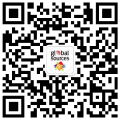“環球資源” 官方微信，獲取即時外貿資訊

# 外貿乾貨 | 如何通過聊天拉近與客戶的距離？

Share:

01

02

03

VOL.1

• hiho=hola=yo=hi=hey=hellow=你好，大家好
• wuz up=sup=what's up=(原意：怎麼樣你？/有什麼事兒嘛？)也可作為問好用(比較熟的兩個人之間的問候)，回答時有事說事，沒事用 "nothing/nothin much/not much/nm" 等回答就可以。

VOL.2

• cya=cu=see ya=see you=再見
• laterz=later=cya later=see ya later=see you later=再見
• gn=gn8=gnight=good night=晚安
• nn=nite=晚安
• 說明：一般第一個人常說 gnight / gn8，然後第二個人用 nite，後面的用 nn 什麼的都可以。

VOL.3

• OMG=oh my god=我的天！
• n1=nice 1=nice one=漂亮
• pwnz=ownz=牛！(例句：pwnz demo!;lefuzee ownz all the others!)
• rullz=強！(例句：lefuzee rullz!;you guyz rull!!!)
• you rock!=你牛！(口語中常用，irc中偶爾能看到)

VOL.4

• lol=laughing out loud /laugh out loud=大笑
• lmao=laughing my ass off=笑翻了
• rofl=roll on floor laughing=笑翻天了

VOL.5

• brb=be right back=馬上回來(例句：gettin some food to eat,brb)
• bbs=be back soon=很快回來
• bbl=be back later=過會兒回來(例句：im gonna go out for a while,bbl)

VOL.6

• gimme=give me=給我
• xfer=transfer=傳輸
• em=them=他們的賓格
• thx=thanks=謝謝
• happy bday=happy b-day=happy birthday!=生日快樂
• dunno=dont know=不知道
• kinda=a little bit=有點(例句：The game is kinda hard for me.i kinda think i should get it done as soon as possible.)
• tho=though=雖然儘管(例句：That demo was very nice,kinda old tho.那個demo很不錯，雖然有點老)
• cmon=c'mon=come on
• enuff=enough=足夠
• hax=hack=cheat=作弊，說謊(很地道時尚的詞，老外用的比較多)
• ty=thank you
• conn=connection=線路,網路,網路類型 (例句:what conn do you have? / cable )
• asap=as soon as possible=儘快
• imo=in my opinion=我認為
• dayum=damn=dang
• ttyl=talk to you later
• b/c=because
• j/j=j/k=j/p=just joking, kidding, playing (開玩笑)
• w/=with
• w/o=without
• holla=來和我講話，also, holla at yo boy, holla at yo girl etc.
• atcha=at you

* 連絡人

* 聯繫電話

• Feedback